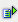The function sqrt (num) calculates the square root of a number.

Syntax: =sqrt(num)Example =sqrt(1) is 1 =sqrt(2) is 1.414214 =sqrt(3) is 1.732051 =sqrt(2) is 1.414214